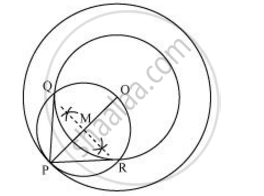# Draw two concentric circles of radii 3 cm and 5 cm. Construct a tangent to smaller circle from a point on the larger circle. Also measure its length. - Mathematics

Draw two concentric circles of radii 3 cm and 5 cm. Construct a tangent to smaller circle from a point on the larger circle. Also measure its length.

#### Solution

Following are the steps to draw tangents on the given circle:

Step 1

Draw a circle of 3 cm radius with centre O on the given plane.

Step 2

Draw a circle of 5 cm radius, taking O as its centre. Locate a point P on this circle and join OP.

Step 3

Bisect OP. Let M be the midpoint of PO.

Step 4

Taking M as its centre and MO as its radius, draw a circle. Let it intersect the given circle at points Q and R.

Step 5

Join PQ and PR. PQ and PR are the required tangents.It can be observed that PQ and PR are of length 4 cm each.

In ΔPQO,

Since PQ is a tangent,

∠PQO = 90°

PO = 5 cm

QO = 3 cm

Applying Pythagoras theorem in ΔPQO, we obtain

PQ2 + QO2 = PQ2

PQ2 + (3)2 = (5)2

PQ2 + 9 = 25

PQ= 25 − 9

PQ= 16

PQ = 4 cm

Hence justified.

Concept: Construction of Tangents to a Circle
Is there an error in this question or solution?
Chapter 9: Constructions - Exercise 9.3 [Page 18]

#### APPEARS IN

RD Sharma Class 10 Maths
Chapter 9 Constructions
Exercise 9.3 | Q 7 | Page 18
Share